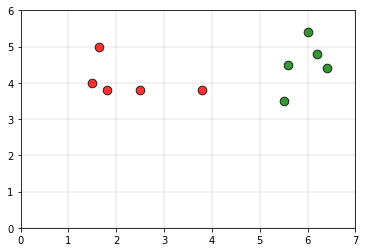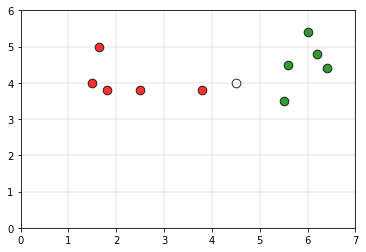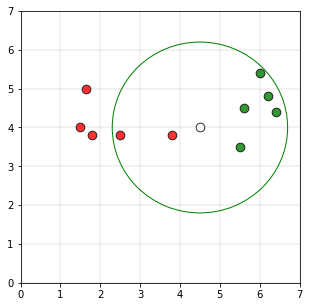# r-Nearest neighbors

r-Nearest neighbors is a modified version of the k-nearest neighbors. The issue with k-nearest neighbors is the choice of k. A smaller k, the classifier would be more sensitive to outliers. If the value of k is large, then the classifier would be including many points from other classes. It is from this logic that we get the r near neighbors algorithm.

Intuition:
Consider the following data, as the training set.The green color points belong to class 0 and the red color points belong to class 1.
Consider the white point P as the query point whoseIf we take the radius of the circle is 2.2 units and if a circle is drawn using the point P as the center of the circle, the plot would be as followsAs the number of points in the circle belonging to class 1 (5 points) are greater than the number of points belonging to class 0 (2 points)

Algorithm:

Step 1: Given the point P, determine the sub-set of data that lies in the ball of radius r centered at P,

Br (P) = { Xi ∊ X | dist( P, Xi ) ≤ r }

Step 2: If Br (P) is empty, then output the majority class of the entire data set.

Step 3: If Br (P) is not empty, output the majority class of the data points in it.

Implementation of the r radius neighbors algorithm is as follows::

## C/C++

 `// C++ program to implement the ` `// r nearest neighbours algorithm. ` `#include ` `using` `namespace` `std; ` ` `  `struct` `Point ` `{ ` `    ``// Class of point ` `    ``int` `val;  ` `     `  `    ``// Co-ordinate of point ` `    ``double` `x, y;  ` `}; ` ` `  `// This function classifies the point p using ` `// r k neareast neighbour algorithm. It assumes only ` `// two groups and returns 0 if p belongs to class 0, else ` `// 1 (belongs to class 1). ` `int` `rNN(Point arr[], ``int` `n, ``float` `r, Point p) ` `{ ` `    ``// frequency of group 0 ` `    ``int` `freq1 = 0;  ` `    ``// frequency of group 1 ` `    ``int` `freq2 = 0;  ` ` `  `    ``// Check if the distance is less than r ` `    ``for` `(``int` `i = 0; i < n; i++) ` `    ``{ ` ` `  `        ``if` `((``sqrt``((arr[i].x - p.x) * (arr[i].x - p.x) +  ` `        ``(arr[i].y - p.y) * (arr[i].y - p.y))) <= r) ` `        ``{ ` `            ``if` `(arr[i].val == 0) ` `                ``freq1++; ` `            ``else` `if` `(arr[i].val == 1) ` `                ``freq2++; ` `        ``} ` `    ``} ` `    ``return` `(freq1 > freq2 ? 0 : 1); ` `} ` ` `  `// Driver code ` `int` `main() ` `{ ` `    ``// Number of data points ` `    ``int` `n = 10;  ` `    ``Point arr[n]; ` ` `  `    ``arr.x = 1.5; ` `    ``arr.y = 4; ` `    ``arr.val = 0; ` ` `  `    ``arr.x = 1.8; ` `    ``arr.y = 3.8; ` `    ``arr.val = 0; ` ` `  `    ``arr.x = 1.65; ` `    ``arr.y = 5; ` `    ``arr.val = 0; ` ` `  `    ``arr.x = 2.5; ` `    ``arr.y = 3.8; ` `    ``arr.val = 0; ` ` `  `    ``arr.x = 3.8; ` `    ``arr.y = 3.8; ` `    ``arr.val = 0; ` ` `  `    ``arr.x = 5.5; ` `    ``arr.y = 3.5; ` `    ``arr.val = 1; ` ` `  `    ``arr.x = 5.6; ` `    ``arr.y = 4.5; ` `    ``arr.val = 1; ` ` `  `    ``arr.x = 6; ` `    ``arr.y = 5.4; ` `    ``arr.val = 1; ` ` `  `    ``arr.x = 6.2; ` `    ``arr.y = 4.8; ` `    ``arr.val = 1; ` ` `  `    ``arr.x = 6.4; ` `    ``arr.y = 4.4; ` `    ``arr.val = 1; ` ` `  `    ``// Query point ` `    ``Point p; ` `    ``p.x = 4.5; ` `    ``p.y = 4; ` ` `  `    ``// Parameter to decide the class of the query point ` `    ``float` `r = 2.2; ` `    ``printf``(``"The value classified to query point"` `           ``" is: %d.\n"``, rNN(arr, n, r, p)); ` `    ``return` `0; ` `} `

## Python3

 `# Python3 program to implement the  ` `# r nearest neighbours algorithm.  ` `import` `math  ` ` `  `def` `rNN(points, p, r ``=` `2.2``):  ` `        ``'''  ` `        ``This function classifies the point p using  ` `        ``r k neareast neighbour algorithm. It assumes only   ` `        ``two groups and returns 0 if p belongs to class 0, else  ` `        ``1 (belongs to class 1).  ` ` `  `        ``Parameters -  ` `                ``points : Dictionary of training points having two ` `                         ``keys - 0 and 1. Each class have a list of ` `                         ``training data points belonging to them  ` ` `  `                ``p : A tuple, test data point of form (x, y)  ` `                ``k : radius of the r nearest neighbors  ` `        ``'''` ` `  `        ``freq1 ``=` `0` `        ``freq2 ``=` `0` `        ``for` `group ``in` `points:  ` `                ``for` `feature ``in` `points[group]:  ` `                        ``if` `math.sqrt((feature[``0``]``-``p[``0``])``*``*``2` `+`  `                                     ``(feature[``1``]``-``p[``1``])``*``*``2``) <``=` `r: ` `                                ``if` `group ``=``=` `0``: ` `                                        ``freq1 ``+``=` `1`                       `                                ``elif` `group ``=``=` `1``:  ` `                                        ``freq2 ``+``=` `1`  `                         `  `        ``return` `0` `if` `freq1>freq2 ``else` `1` ` `  `# Driver function  ` `def` `main():  ` ` `  `        ``# Dictionary of training points having two keys - 0 and 1  ` `        ``# key 0 have points belong to class 0  ` `        ``# key 1 have points belong to class 1  ` ` `  `        ``points ``=` `{``0``:[(``1.5``, ``4``), (``1.8``, ``3.8``), (``1.65``, ``5``), (``2.5``, ``3.8``), (``3.8``, ``3.8``)],  ` `                  ``1``:[(``5.5``, ``3.5``), (``5.6``, ``4.5``), (``6``, ``5.4``), (``6.2``, ``4.8``), (``6.4``, ``4.4``)]}  ` ` `  `        ``# query point p(x, y)  ` `        ``p ``=` `(``4.5``, ``4``)  ` ` `  `        ``# Parameter to decide the class of the query point  ` `        ``r ``=` `2.2` ` `  `        ``print``(``"The value classified to query point is: {}"``.``format``( ` `                ``rNN(points, p, r)))  ` ` `  `if` `__name__ ``=``=` `'__main__'``:  ` `        ``main()  `

Output:

```The value classified to query point is: 1.
```

Other techniques like kd-tree, locality sensitive hashing can be used to reduce the time complexity of finding the neighbors.

Applications:
This algorithm can be used to identify outliers. If a pattern does not have any similarity with the patterns within the radius chosen, it can be identified as an outlier.

GeeksforGeeks has prepared a complete interview preparation course with premium videos, theory, practice problems, TA support and many more features. Please refer Placement 100 for details

My Personal Notes arrow_drop_upCheck out this Author's contributed articles.

If you like GeeksforGeeks and would like to contribute, you can also write an article using contribute.geeksforgeeks.org or mail your article to contribute@geeksforgeeks.org. See your article appearing on the GeeksforGeeks main page and help other Geeks.

Please Improve this article if you find anything incorrect by clicking on the "Improve Article" button below.